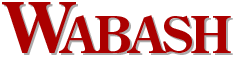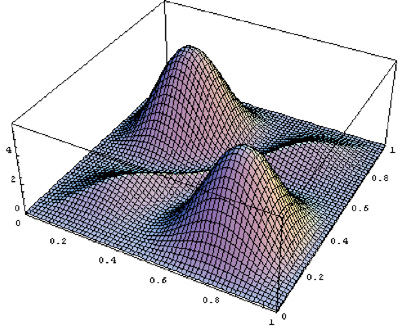"An equation is a work of art if it is simple, can be solved exactly in terms of well-known functions, gives rise to a picture, is filled with information, has an expression of symmetry, and teaches us something new."Magazine Winter/Spring 2002 A pursuit of beauty by Paul McKinney ’52 The equation of motion for particle systems, especially in the world of atoms and molecules, is a pursuit of beauty—quantum mechanics, it is called. An equation is a work of art if it is simple, can be solved exactly in terms of well-known functions, gives rise to a picture, is filled with information, has an expression of symmetry, and teaches us something new. Here is an example of one of my recent quantum reveries: Suppose you have two deltons, my name for some as yet unknown and undiscovered particles, moving on a straight wire of length L. Usually the deltons ignore each other, are unaware of each other’s existence; however, if they occupy the same position, they repel each other infinitely. Erwin Schrödinger showed us how to find the probability density functions and the energies for such wave-particle systems in the 1930s. A probability density function is like a photograph—a snapshot of the deltons if we take their picture over and over again. For this system, it is not possible to separate out the motion of each individual delton, but, interestingly enough, I can solve the equation exactly for the two particle system using well-known trigonometry functions, sines, and applying the system’s boundary conditions, I calculate an infinite set of discrete energy levels. Mathematica, a computer program which transforms equations into beautiful pictures, can plot two different views of probability density functions: Three-dimensional graphs and contour maps. Selecting just one energy level, I discover two probability density functions—I only expected one!Look: the graph has four elements of symmetry! The identity, two planes of reflection along the diagonals of the square, and a 180 degree axis of rotation about the center of the square. The hills on the 3-D graphs tell the most probable places to find the deltons. Even though the deltons repel each other infinitely when they are at the same position, there is a finite probability that they will occupy the same position according to the graphs. Strange, but exciting! Now the door to the problem’s information is open! My curiosity is piqued! A feeling of pleasure grips me! The initial result’s beauty stimulates me to explore the problem’s landscape further! Paul McKinney is professor of chemistry and dean emeritus at Wabash. Return to INCENSE Return to the table of contents